# JEE Main 2020 Maths Paper With Solutions Shift 1 Sept 3

JEE Main 2020 Maths paper September 3 Shift 1 solutions are available on this page. These are solved by specialized experts at BYJU’S. Learning these problems will help students to get an idea of the difficulty level of the JEE Main exam. Students are advised to practise sample papers so that they can be confident and stress-free during the exam. Aspirants can easily download the PDF format of these solutions for free.

### September 3 Shift 1 - Maths

1. The value of (2.1P0-3.2P1+4.3P2-..... up to 51th term) +(1!-2!+3!-...... up to 51th term) is equal to:

1. a) 1-51(51)!
2. b) 1+(52)!
3. c) 1
4. d) 1+ (51)!

Solution:

1.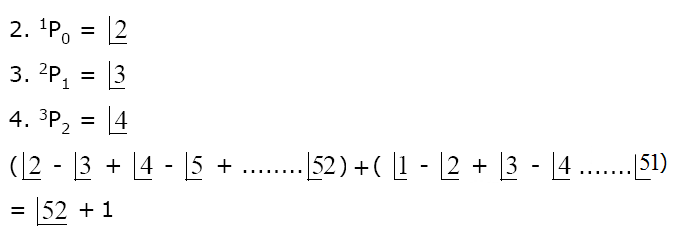2. Let P be a point on the parabola, y2 = 12x and N be the foot of the perpendicular drawn from P on the axis of the parabola. A line is now drawn through the mid-point M of PN, parallel to its axis which meets the parabola at Q. If the y-intercept of the line NQ is 4/3 , then:

1. a) PN = 4
2. b) MQ = 1/3
3. c) PN = 3
4. d) MQ = 1/4

Solution:

1.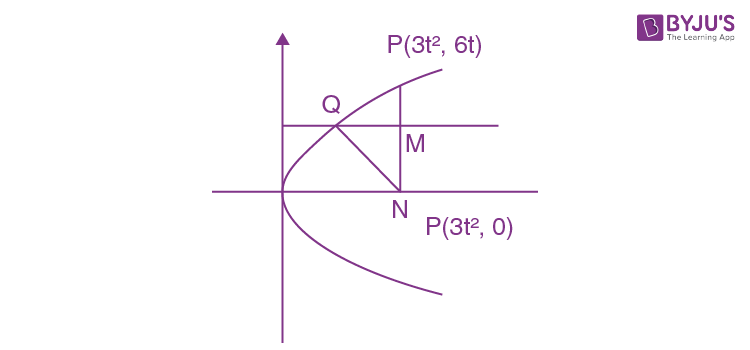Q (h, 3t) lie on parabola 9t2 = 12h

h = 3t2/4

Q = (3t2/4, 3t)

Equation of NQ =

y = 3t(x-3t2)/((3t2/4)-3t2)

y = -4(x-3t2)/3t

put x = 0

y = 4t

4t = 4/3

⇒ t = 1/3

PN = 6t

= 6×(1/3)

= 2

M = (1/3, 1), Q = (1/12,1)

MQ = (1/3)-(1/12)

MQ = 1/4

3. If Δ =

$$\begin{vmatrix} x-2 & 2x-3 & 3x-4\\ 2x-3&3x-4 & 4x-5\\ 3x-5 & 5x-8 &10x-17 \end{vmatrix}$$
= Ax3+Bx2+Cx+D, then B+C is equal to:

1. a) 1
2. b) -1
3. c) -3
4. d) 9

Solution:

1. $$\begin{vmatrix} x-2 & 2x-3 & 3x-4\\ 2x-3&3x-4 & 4x-5\\ 3x-5 & 5x-8 &10x-17 \end{vmatrix}$$
= Ax3+Bx2+Cx+D

R2 ⇒ R2 -R1

R3 ⇒ R3 -R2

$$\begin{vmatrix} x-2 & 2x-3 & 3x-4\\ x-1&x-1 & x-1\\ x-2 & 2(x-2) &6(x-2) \end{vmatrix}$$
= Ax3+Bx2+Cx+D

$$(x-1)(x-2)\begin{vmatrix} x-2 & 2x-3 & 3x-4\\ 1&1 & 1\\ 1 & 2 &6 \end{vmatrix}$$
= Ax3+Bx2+Cx+D

⇒ (x-1)(x-2){(x-2)(6- 2)-(2x-3)(6-1)+(3x- 4)(2-1)} = Ax3+Bx2+Cx+D

⇒ (x-1)(x -2){4x-8 -10x+15+3x-4} = Ax3+Bx2+Cx+D

⇒ (x-1)(x-2)(-3x +3) = Ax3+Bx2+Cx+D

-3(x-1)2 (x-2) = Ax3+Bx2+Cx+D

-3x3 +12x2-15x+6 = Ax3+Bx2+Cx+D

Comparing both side

A = -3, B = 12, C = -15

B+C = 12-15 = -3

4. The foot of the perpendicular drawn from the point (4,2,3) to the line joining the points (1,2,3) and (1,1,0) lies on the plane:

1. a) x-y-2z = 1
2. b) x-2y+z = 1
3. c) 2x+y-z = 1
4. d) x+2y-z = 1

Solution:

1. $$\vec{r} = (1,-2,3)+\lambda (0,3,-3)$$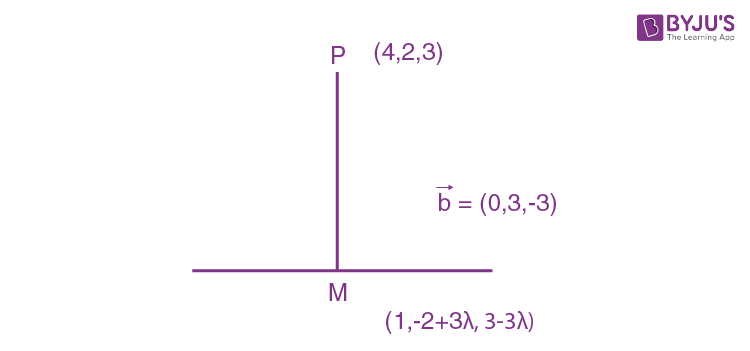$$\overrightarrow{PM} ⊥ \overrightarrow{b}$$

$$\overrightarrow{PM} . \overrightarrow{b}= 0$$

(-3, 3λ-4, -3λ).(0,3, -3) = 0

⇒ 0+9λ-12+9λ = 0

⇒ λ= 12/18 = 2/3

m = (1,0,1) are on 2x+y-z = 1

5. If y2+loge(cos2x) = y, x∈(-π/2, π/2), then

1. a) |y’(0)|+|y’’(0)| = 1
2. b) |y’’(0)| = 0
3. c) |y’(0)|+|y’’(0)| = 3
4. d) |y’’(0)| = 2

Solution:

1. yy’ + 2 (-tanx) = y’ ....(1)

diff. w.r.t.x

2y’’+2(y’)2 -2 sec2 x = y” ....(2)

Put x = 0 in given equation we get y = 0, 1

from (1) x = 0, y = 0

⇒ y’(0) = 0

x = 0, y = 1

⇒ y’(0) = 0

from (2) x = 0, y = 0, y’(0) = 0 ⇒ y”(0) = -2

x = 0, y = 1, y’(0) = 0 ⇒ y”(0) = 2

|y”(0)| = 2

6. 2π-(sin-1(4/5)+sin-1(5/13)+sin-1(16/65)) is equal to:

1. a) 5π/4
2. b) 3π/2
3. c) 7π/4
4. d) π/2

Solution:

1. 2π-(sin-1(4/5)+sin-1(5/13)+sin-1(16/65)) = 2π-(tan-1(4/3)+tan-1(5/12)+tan-1(16/63))

= 2π-tan-1 [(4/3)+(5/12)/(1-(4/3)(5/12) ]- tan-1(16/63)

= 2π-tan-1(48+15)/(36-20) - tan-1(16/63)

= 2π-tan-1(63/16) - cot-1(63/16)

= 2π-π/2

= 3π/2

7. A hyperbola having the transverse axis of length √2 has the same foci as that of the ellipse 3x2+4y2 = 12, then this hyperbola does not pass through which of the following points ?

1. a) (√(3/2), 1/√2)
2. b) (1, -1/√2)
3. c) (1/√2, 0)
4. d) (-√(3/2),1)

Solution:

1. (x2/4)+(y2/3) = 1

b12 = a12(1-e12)

3 = 4(1-e12)

e1 = 1/2

focus = (± a1e1, 0)

= (±1, 0)

Length of the transverse axis 2a2 = √2

a2 = 1/√2

a2e2 = 1

So e2 = √2

b22 = a22(e22-1)

b22 = (1/2)(2-1)

b22 = 1/2

Equation of hyperbola is x2-y2 = 1/2.

(√(3/2), 1/√2) does not lie on it.

8. For the frequency distribution

Variate (X): x1 x2 x3…x15

Frequency (f): f1 f2 f3…f15

where 0<x1<x2< x3<… x15 ≤ 10 and

$$\sum_{i=1}^{15}f_{i}> 0$$
, the standard deviation cannot be:

1. a) 1
2. b) 4
3. c) 6
4. d) 2

Solution:

1. σ2 ≤ (1/4)(M-m)2

M = upper bound of value of any random variable

m = Lower bound of value of any random variable

σ2 ≤ (1/4)(10-0)2

σ2 ≤ 25

-5< σ < 5

σ ≠ 6

9. A die is thrown two times and the sum of the scores appearing on the die is observed to be a multiple of 4. Then the conditional probability that the score 4 has appeared atleast once is:

1. a) 1/3
2. b) 1/4
3. c) 1/8
4. d) 1/9

Solution:

1. Total Possibilities = (1, 3), (3, 1), (2, 2), (2, 6), (6, 2) (4, 4), (3, 5), (5, 3) (6, 6)

(A ∩ B) = (4,4)

n(A ∩ B) = 1

Required probability = P(B/A)

= P(A ∩ B)/P(A)

= 1/9

10. If the number of integral terms in the expansion of (31/2 +51/8)n is exactly 33, then the least value of n is:

1. a) 128
2. b) 248
3. c) 256
4. d) 264

Solution:

1. Tr+1 = nCr(31/2)n-r(51/8)r

(n-r)/2 -> n-r = 0, 2, 4, 6, 8,…

r/8 -> r = 0, 8, 16, 24….

common ratio = 0, 8, 16, 24.......

no. of integral term = 33.

L = 0+(33-1)×8

L = 32×8

= 256

11.

$$\int_{-\pi }^{\pi }\left | \pi -\left | x \right | \right |dx$$
is equal to:

1. a) π2
2. b) π2/2
3. c) √2π2
4. d) 2π2

Solution:

1. $$\int_{-\pi }^{\pi }\left | \pi -\left | x \right | \right |dx$$
even function

$$2\int_{0 }^{\pi }\left | \pi -x \right |dx$$

= 2[πx-x2/2]0π

= 2[π2/2]

= π2

12. Consider the two sets:

A = {m ∈ R : both the roots of x2-(m+1)x+m+4 = 0 are real} and B = [-3,5).

Which of the following is not true ?

1. a) A-B = (-∞,-3) ∪ (5,∞)
2. b) A ∩ B = {-3}
3. c) B-A = (-3,5)
4. d) A U B = R

Solution:

1. D ≥ 0

(m+1)2 -4(m+4) ≥ 0

m2-2m-15 ≥ 0

(m-5)(m+3) ≥ 0

m ∈(-∞, -3] U [5, ∞)

A = (-∞, -3] U [5, ∞)

B = [-3, 5]

A-B = (-∞, -3] U [5, ∞)

A U B = R

Only first option is not true.

13. The proposition p -> ∼(p˄q) is equivalent to:

1. a) (∼p) ˅(∼ q)
2. b) (∼ p) ˄q
3. c) q
4. d) (∼ p) ˅q

Solution:

1. ∼(p˄ ∼q) → ∼ p˅q

p → (p∼˅q)

⇒ ∼ p˅(∼p˅q)

⇒ ∼ p˅q

14. The function, f(x) = (3x-7)x2/3, x ∈ R is increasing for all x lying in:

1. a) (-∞, -14/15) U (0,∞)
2. b) (-∞, 14/15)
3. c) (-∞,0)U(14/15, ∞)
4. d) (-∞,0)U(3/7, ∞)

Solution:

1. f’(x) = (3x-7)(2/3x1/3)+(x2/3×3)

= (6x-14+9x)/3x1/3

= (15x-14)/3x1/3f(x) >0 ⇒ x ∈ (-∞,0)U(14/15, ∞)

15. If the first term of an A.P. is 3 and the sum of its first 25 terms is equal to the sum of its next 15 terms, then the common difference of this A.P. is:

1. a) 1/6
2. b) 1/5
3. c) 1/4
4. d) 1/7

Solution:

1. a = 3

(25/2)(2a+24d) = (15/2)(2(a+25d)+14d)

50a+600d = 15[2a+50d+14d]

20a+600d = 960d

60 = 360d

d = 1/6

16. The solution curve of the differential equation, (1+e-x)(1+y2)dy/dx = y2, which passes through the point (0,1), is:

1. a) y2 = 1+y loge(1+e-x)/2
2. b) y2 +1 = y ((loge(1+e-x)/2 )+2)
3. c) y2 +1 = y ((loge(1+ex)/2 )+2)
4. d) y2 = 1+y loge(1+ex)/2

Solution:

1. $$\int \left ( \frac{1+y^{2}}{y^{2}} \right )dy=\int \left ( \frac{1}{1+e^{-x}} \right )dx$$

$$\int \frac{1}{y^{2}}dy+\int dy=\int \frac{e^{x}}{e^{x}+1}dx$$

⇒ (-1/y)+y = lnex+1 + C

x = 0, y = 1

⇒ -1+1 = ln 2+C

⇒ C = -ln 2

⇒ (-1/y)+y = lnex+1 - ln 2

⇒ y2 = 1+y[ln(ex+1)/2]

17. The area (in sq. units) of the region {(x,y):0 ≤y ≤x2+1, 0 ≤y ≤x+1, 1/2 ≤x≤2} is

1. a) 23/16
2. b) 79/16
3. c) 23/6
4. d) 79/24

Solution:

1.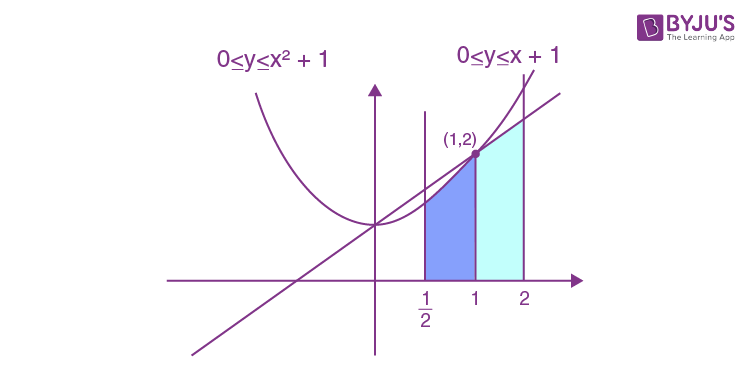A=

$$\int_{\frac{1}{2}}^{1}(x^{2}+1)dx+\int_{1}^{2}(x+1)dx$$

=

$$\left ( \frac{x^{3}}{3}+x\right )_{\frac{1}{2}}^{1}+\left ( \frac{x^{2}}{2}+x \right )_{1}^{2}$$

= (1/3)+1-[(1/24)+(1/2)]+[2+2-(3/2)]

= (4/3)-(13/24)+(5/2)

= (19+60)/24

= 79/24

18. If α and β are the roots of the equation x2+px+2 = 0 and (1/α) and (1/β) are the roots of the equation 2x2+2qx+1 = 0, then (α-1/α)(β-1/β)(α+1/α)(β+1/β) is equal to :

1. a) (9/4)(9+p2)
2. b) (9/4)(9+q2)
3. c) (9/4)(9-p2)
4. d) (9/4)(9-q2)

Solution:

1. α+β = -p

αβ = 2

(1/α)+(1/β) = -q

1/αβ = 1/2

(α+1/β)(β+1/α) = αβ+(1/αβ)+2

= 2+(1/2)+2

= 9/2

(α-1/α)(β-1/β) = αβ+(1/αβ)-(α/β)-(β/α)

= 2+(1/2)-(α22)/αβ

= (5/2)-[((α+β)2-2αβ)/αβ)]

= (5/2)-[(p2-4)/2]

= (9-p2)/2

(α-1/α)(β-1/β)(α+1/α)(β+1/β) = ((9-p2)/2)(9/2)

= (9/4)(9-p2)

19. The lines

$$\vec{r}= (\hat{i}-\hat{j})+l(2\hat{i}+\hat{k})$$
and
$$\vec{r}= (2\hat{i}-\hat{j})+m(\hat{i}+\hat{j}-\hat{k})$$

1. a) do not intersect for any values of l and m
2. b) intersect when l = 1 and m = 2
3. c) intersect when l = 2 and m = 1/2
4. d) intersect for all values of l and m

Solution:

1. $$\vec{r}= (\hat{i}-\hat{j})+l(2\hat{i}+\hat{k})$$
..(i)

$$\vec{r}= (2\hat{i}-\hat{j})+m(\hat{i}+\hat{j}-\hat{k})$$
…(ii)

Let two lines

$$\vec{r}= \vec{a}+t\vec{b}$$

and

$$\vec{r}= \vec{c}+t\vec{d}$$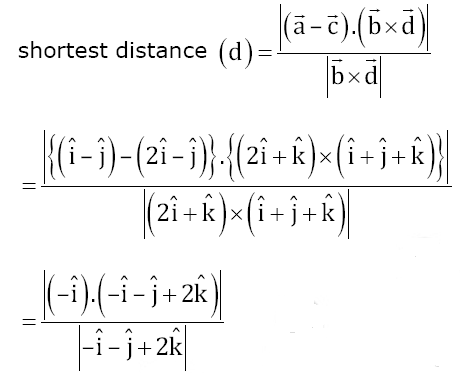= 1/√(-1)2+(-1)2+22

= 1/√6

Since shortest distance between these lines exist

Hence lines do not intersect.

20. Let [t] denote the greatest integer ≤t. If for some λ ∈ R -{0, 1},

$$\lim_{x\to0}\left | \frac{1-x+\left | x \right |}{\lambda -x+[x]} \right |= L$$

Then L is equal to:

1. a) 0
2. b) 2
3. c) 1/2
4. d) 1

Solution:

1. $$\lim_{x\to0}\left | \frac{1-x+\left | x \right |}{\lambda -x+[x]} \right |= L$$

RHL:

$$\lim_{x\to0^{+}}\left | \frac{1-x+x}{\lambda -x+[x]} \right |$$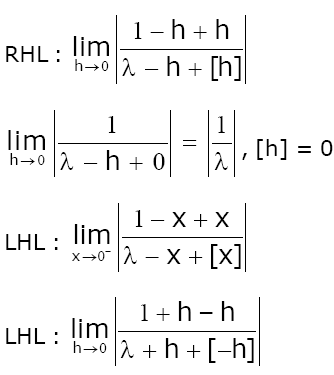= 1/(-1)

Since |λ| = |λ-1|, [-h] = -1

λ2 = λ2-2λ+1

⇒ λ= 1/2

L = 2

21. If

$$\lim_{x\to0}\left \{ \frac{1}{x^{8}}\left ( 1-\cos \frac{x^{2}}{2} -\cos \frac{x^{2}}{4}+\cos \frac{x^{2}}{2}\cos \frac{x^{2}}{4}\right ) \right \}= 2^{-k}$$
then the value of k is:

Solution:

1. $$\lim_{x\to0}\frac{(1-\cos \frac{x^{2}}{2})(1-\cos \frac{x^{2}}{4})(\frac{x^{2}}{2})^{2}(\frac{x^{2}}{4})^{2}}{(\frac{x^{2}}{2})^{2}(\frac{x^{2}}{4})^{2}x^{8}}$$

$$\lim_{x\to0}\frac{1}{4}\times \frac{1}{4}\times \frac{1}{16}$$

⇒ 1/256 = 2-k

⇒ 2-8 = 2-k

⇒ k = 8

22. The diameter of the circle, whose centre lies on the line x+y = 2 in the first quadrant and which touches both the lines x = 3 and y = 2, is ......

Solution:

1.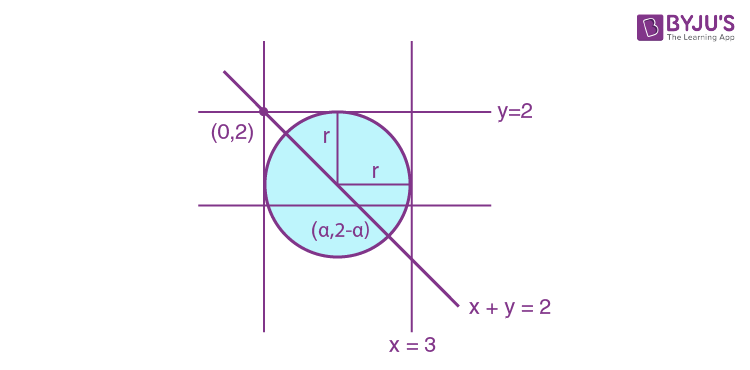p = r

for y = 2

r = |(2-α-2)/1| = |α|

for x = 3

r = |(α-3)/1| = |α-3|

| α | = |α - 3 |

⇒ α2 = α2-6α+9

⇒ α = 3/2

2α = 3 = 2r

23. The value of

$$0.16^{log_{2.5}\frac{1}{3}+\frac{1}{3^{2}}+\frac{1}{3^{3}}+...\infty }$$
is equal to:

Solution:

1. (1/3)+(1/32)+(1/33)+….∞ = 1/3(1-1/3) = 1/2

log2.5(1/2) ⇒ log5/2 (1/2)

0.16 = 16/100 = 4/25 = (2/5)2

$$\left (\frac{2}{5} \right )^{2log_{\frac{5}{2}}\frac{1}{2}} = \left (\frac{5}{2} \right )^{-2log_{\frac{5}{2}}\frac{1}{2}}$$

$$\left (\frac{5}{2} \right )^{log_{\frac{5}{2}}(\frac{1}{2})^{-2}}$$

= 4

24. Let A =

$$\begin{bmatrix} x &1 \\ 1 & 0 \end{bmatrix}$$
, x∈R and A4 = [aij]. If a11 = 109, then a22 is equal to:

Solution:

1. A =

$$\begin{bmatrix} x &1 \\ 1 & 0 \end{bmatrix}$$

A2 =

$$\begin{bmatrix} x &1 \\ 1 & 0 \end{bmatrix}\begin{bmatrix} x &1 \\ 1 & 0 \end{bmatrix}$$
=
$$\begin{bmatrix} x^{2} +1&x\\ x & 1 \end{bmatrix}$$

A3 =

$$\begin{bmatrix} x^{2} +1&x\\ x & 1 \end{bmatrix}\begin{bmatrix} x &1 \\ 1 & 0 \end{bmatrix}$$

=

$$\begin{bmatrix} x^{3} +x+x&x^{2}+1\\ x ^{2}+1& x \end{bmatrix}$$

A4 =

$$\begin{bmatrix} x^{3} +2x&x^{2}+1\\ x ^{2}+1& x \end{bmatrix}\begin{bmatrix} x &1 \\ 1 & 0 \end{bmatrix}$$

=

$$\begin{bmatrix} x^{4} +2x^{2}+x^{2}+1&x^{3}+2x\\ x ^{3}+x+x& x^{2} +1\end{bmatrix}$$

a11 ⇒ x4+3x2+1 = 109

x4 +3x2-108 = 0

⇒ (x2+12)(x2-9) = 0

x = ±3

a22 = x2+1 = 10

25. If ((1+i)/(1-i))m/2 = ((1+i)/(i-1))n/3 = 1, (m, n∈N) then the greatest common divisor of the least values of m and n is :

Solution:

1. [(1+i)(1+i)/(1+i)(1-i)]m/2 = [(1+i)(-1-i)/(-1+i)(-1-i)]n/3 = 1 (i = √-1)

Solving LHS of above equation, we get

(2i/2)m/2 = 1

⇒ m = 8

Solving RHS,

[(-1-i-i+1)/(1+1)]n/3 = 1

(-2i/2)n/3 = 1

(-i)n/3 = 1

⇒ n = 12

Greatest common divisor of m and n is 4.

### JEE Main 2020 Maths Paper With Solutions Shift 1 September 3